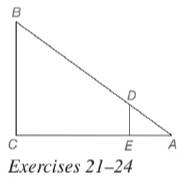Chapter 5.2, Problem 23EElementary Geometry For College St...

7th Edition
Alexander + 2 others
ISBN: 9781337614085

Solutions

Chapter
SectionElementary Geometry For College St...

7th Edition
Alexander + 2 others
ISBN: 9781337614085
Textbook Problem

In Exercises 21 to 24, △ A D E ∼ △ A B C . Given: D E = 4 , A C = 20 , E C = B C Find: B CTo determine

To calculate:

The value of the side BC.

Explanation

Given:

It is given that ΔADEABC.

DE=4,AC=20,andEC=BC

Definition Used:

Similarity between Two polygons:

Two polygons are similar if and only if when all pairs of corresponding angles are congruent and all pairs of corresponding sides are proportional.

If C is a point of XY¯ and XCY, then XC+CY=XY.

Calculation:

According to the definition of similar polygon, ACAE=BCDE=ABAD.

According to the segment-addition postulate, AC=AE+EC.

Still sussing out bartleby?

Check out a sample textbook solution.

See a sample solution

The Solution to Your Study Problems

Bartleby provides explanations to thousands of textbook problems written by our experts, many with advanced degrees!

Get Started

Use Property 8 to estimate the value of the integral. 01x3dx

Single Variable Calculus: Early Transcendentals, Volume I

What does Clairauts Theorem say?

Calculus (MindTap Course List)

Solve the equations in Exercises 126. x69x4=0

Finite Mathematics and Applied Calculus (MindTap Course List)

True or False: is monotonic.

Study Guide for Stewart's Single Variable Calculus: Early Transcendentals, 8th